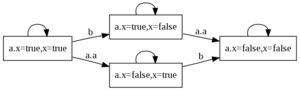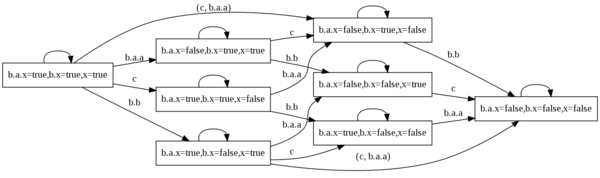# Implicit synchronizations with epsilon

Nodes that do not appear in a synchronization vector are synchronized with the event ε (epsilon). This implicit synchronization can transform events of a node N as hidden events in the ancestor of the parent of N. To illustrate this phenomenon, consider a system with 3 levels of nodes as given below.

``` 1 node A
2   event a;
3   state x : bool;
4   trans x |- a -> x := not x;
5 edon
6
7 node B
8   sub a : A;
9   event b;
10   state x : bool;
11   trans x |- b -> x := not x;
12 edon
13
14 node C
15   sub b : B;
16   event c;
17   state x : bool;
18   trans x |- c -> x := not x;
19 edon```

The semantics of nodes `A` is straightforward (see the figure below).As explained in previous sections, the semantics of `B` represents the interleaving of the event `a` of sub-node `a` and the event `b` of `B`. On the figure below, epsilon events are displayed as empty labels. Actually transitions of `B` should be labelled by synchronization vectors of the node i.e. `<` ε, a.ε `>`, `<` ε, a.a `>` and `<` b, a.ε `>`.Now, consider the case of node `C`. Implicit vectors of `C` are analog to the ones of `B`: `<` ε, b.ε `>`, `<` ε, b.b `>` and `<` c, b. ε `>`. The transitivity of the synchronization implies that the actual synchronization vectors for the whole model are:

• `<` ε, `<` b.b, b.a.ε `> >`
• `<` c, `<` b.ε, b.a.a `> >`
• `<` c, `<` b.ε, b.a.ε `> >`
• `<` ε, `<` b.ε, b.a.a `> >`
• `<` ε, `<` b.ε, b.a.ε `> >`

These vectors yield to the following semantics of the node `C`:# Visibility attributes

AltaRica descriptions must respect some visibility rules applied to variables and events. Default rules are given below:

• A subnode does not known events or variables existing in its parent node. This rule is guaranteed by the order of declaration of nodes.
• A compound node can constrain only flow variables and events of its declared subnodes.
• A compound node can change the initial values of all state variables at any depth in its hierarchy.

Default rules can be bypassed using visibility attributes that can be applied to events or variables:

• `private` means that the object is visible only by the node that has declared it.
• `parent` means that the object is also visible by the parent node.
• `public` means that the object is visible by all ancestors.

Using these attributes, default visibility rules can be summarized as follows: state variables are assigned the `private` attribute (except for initialization), events and flow variables are assigned `parent`.

While `public` variables are visible at the top-level of the AltaRica model hierarchy, the `public` attribute of events is removed at the level of the first explicit synchronization. Example:

``` 1 node A
2   event e : public;
3 edon
4
5 node B
6   sub a : A;
7   event f;
8 edon
9
10 node C
11   sub b1, b2 : B;
12   sync <b1.f, b2.a.e>; // authorized
13 edon```

Synchronized events can not have a common prefix. For instance on the next example, the node `C` can not synchronize `b1.f` and `b1.a.e`; the following synchronization is thus forbidden:

``` 1 node A
2   event e : public;
3 edon
4
5 node B
6   sub a : A;
7   event f;
8   sync <f, a.e>;
9 edon
10
11 node C
12   sub b1, b2 : B;
13   sync <b1.f, b2.a.e>; // not authorized because 'a.e'
14                        // is not an event of the interface of b2.
15 edon```

# Priorities

AltaRica language permits to specify priorities between events. In a given configuration, if two events are possible then only the one having the highest priority can actually occur. Priorities are locally defined in a node by a partial order on events. If two events are incomparable w.r.t the specification then they are both possible.

The declaration of events and the specification of the partial order can be mixed; for the sake of clarity, one should prefer to define the order after the declaration of events.

``````1 node Component;
2   event a, b;
3         a < b; // only this line is actually necessary to declare a and b
4   ...
5 edon
``````

The syntax of partial order uses comparators `<` and `>`. Both sides of these comparators can be either simple events or sets of events enclosed between braces. The following specifications are equivalent:

• `a < c`, `a < d`, `b < c`, `b < d`
• `{ a, b } < c`, `{ a, b } < d`,
• `a < { c, d }`, `b < { c, d }`
• `{ a, b } < { c, d }`

The partial order is applied to the semantics of the node i.e. it takes into account assertions and the hierarchy. Priorities are just a syntactic sugar for leaf nodes because the order can be integrated into guards of transitions. For compound nodes, the order is applied after synchronization of subnodes, thus priorities define in fact an order on
synchronization vector based on events of the hierarchical node. Compound nodes can be viewed as controllers that constrain their subnodes using: assertions, synchronizations and priorities.

# Weak synchronizations

Synchronization vectors defined (implicitly or explicitly) in a node are allowed combination of events. A combination can occur in the system if all components of the vector are possible; thus, if just one of the component is inhibited for some reason the vector can not happen.

Using this synchronization mechanism, the modelling of a broadcast phenomenon or a common failure mode becomes tricky and not natural because if some events are missing the synchronized event can not occur. For this reason, the AltaRica language permits to write weak synchronization vectors (usual vectors are called strong). Syntactically it just consists in adding question-marks (`?`) to events.

The semantics of weak vectors is the following:

• each event marked with a question-mark is not mandatory for the synchronization;
• if a marked event is possible then it should participate to the synchronization;
• a marked event that does not participate to the synchronization is replaced by ε.

In a given configuration, the synchronizations that occur are those that maximize the number of enabled marked events. The way this semantics is formalized is to consider that each weak vector induces a set of strong vectors that encode all the possible combinations of marked events. This set is then partially ordered by the inclusion ordering. For instance, the vector `< a?, b?, c? >` induces the following lattice:Given this set of vectors and the partial order on this set the standard semantics with priorities is applied. Note that the order on vectors is applied prior to the order on local events of the compound nodes.

The language proposes additional notations to manipulate the lattice.

• Constraints on the number of marked events can be specified (> n, < n, >= n, <= n, = n) to enforce a given cardinality of possible marked events. For instance if >= 1 means that at least one marked event has to participate to the synchronization.
• `min` and `max` keywords specified at the end of the vector indicates that the synchronization has to use a minimal or a maximal number of marked events (by default `max` is used).

# Abstract data types

Since ACI Persée, the language supports the use of abstract data types. These types are given after a `sort` keyword followed by a comma-separated list of type identifiers. Example:

``````1 sort MESSAGE, QUEUE;
``````

Once declared, these types can be used as others; in particular abstract constants can be defined:

``````1 const ACK_OK : MESSAGE;
2 const ACK_NOK : MESSAGE;
3 const MSG_DATA : MESSAGE;
``````

In order to manipulate variables and constants defined with an abstract type, signatures of functions can be declared. A signature is introduced by the `sig` keyword followed by the identifier of the function, a colon and input and output types of the function; the input type can be a Cartesian product given as star-separated list of types. Example:

``````1 sig push : QUEUE * MESSAGE -> QUEUE;
2 sig top : QUEUE -> MESSAGE;
3 sig pop : QUEUE -> QUEUE;
4 sig is_empty : QUEUE -> bool;
``````

Abstract types, constants and signatures can be used in any AltaRica expression. Up to now only ARC supports this extension and this support is limited to the flat semantics of nodes.